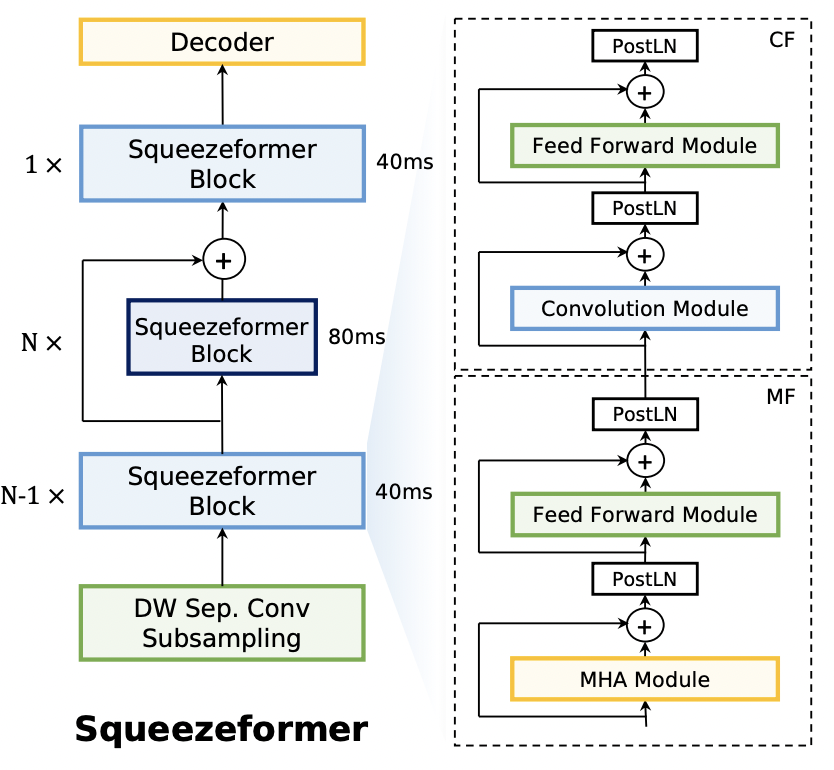# Squeezeformer

Squeezeformer incorporates the Temporal U-Net structure, which reduces the cost of the multi-head attention modules on long sequences, and a simpler block structure of feed-forward module, followed up by multi-head attention or convolution modules, instead of the Macaron structure proposed in Conformer.This repository contains only model code, but you can train with squeezeformer at openspeech.

## Installation

``````pip install squeezeformer
``````

## Usage

```import torch
import torch.nn as nn
from squeezeformer.model import Squeezeformer

BATCH_SIZE = 4
SEQ_LENGTH = 500
INPUT_SIZE = 80
NUM_CLASSES = 10

device = torch.device('cuda' if torch.cuda.is_available() else 'cpu')

criterion = nn.CTCLoss().to(device)
model = Squeezeformer(
num_classes=NUM_CLASSES,
).to(device)

inputs = torch.FloatTensor(BATCH_SIZE, SEQ_LENGTH, INPUT_SIZE).to(device)
input_lengths = torch.IntTensor([500, 450, 400, 350]).to(device)
targets = torch.LongTensor([[1, 3, 3, 3, 3, 3, 4, 5, 6, 2],
[1, 3, 3, 3, 3, 3, 4, 5, 2, 0],
[1, 3, 3, 3, 3, 3, 4, 2, 0, 0],
[1, 3, 3, 3, 3, 3, 6, 2, 0, 0]]).to(device)
target_lengths = torch.LongTensor([9, 8, 7, 7]).to(device)

# Forward propagate
outputs, output_lengths = model(inputs, input_lengths)

# Calculate CTC Loss
for _ in range(3):
outputs, output_lengths = model(inputs, input_lengths)
loss = criterion(outputs.transpose(0, 1), targets[:, 1:], output_lengths, target_lengths)
loss.backward()```

## Reference

``````Copyright 2022 Sangchun Ha.

you may not use this file except in compliance with the License.
You may obtain a copy of the License at